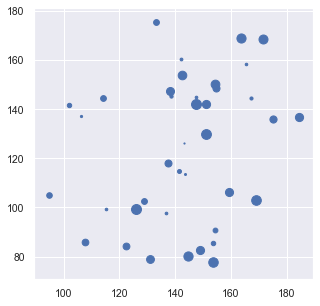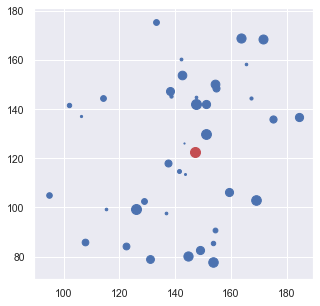# Notebook 5.1 - Continuous Facility Location¶

In :
import numpy as np
import matplotlib
import matplotlib.pyplot as plt
import seaborn as sns; sns.set()
from sklearn.datasets import make_blobs

%matplotlib inline

plot_size   = 14
plot_width  = 5
plot_height = 5

params = {'legend.fontsize': 'large',
'figure.figsize': (plot_width,plot_height),
'axes.labelsize': plot_size,
'axes.titlesize': plot_size,
'xtick.labelsize': plot_size*0.75,
'ytick.labelsize': plot_size*0.75,
plt.rcParams.update(params)


As before, we are importing the packages tht we are going to use, and prepare the properties of the plots.

In :
num_customers = 40

center_box = (100, 200) # defines the box that cluster centres are allowed to be in

coord, clust_true = make_blobs(n_samples=num_customers,
centers=2,
cluster_std=20,
center_box=center_box,
random_state = 2)

weights = list(np.random.uniform(size=len(coord)))

for i in range(len(coord)):
plt.scatter(coord[i, 0], coord[i, 1], s=weights[i]*100, c='b');We will use the make_blobs command again to generate a set of customers. What we do differently here, however, is to to define a centre_box vector, that forces the generator to position points around a different point (the default value is 0,0).

In :
import csv

with open("flp_coords.csv","w+") as my_csv:
csvWriter = csv.writer(my_csv,delimiter=',')
csvWriter.writerows(coord)


Using the csv package, we are saving the coordinates to a csv file (flp_coords.csv) in the same directory.

In :
from cvxpy import Minimize, Problem, CVXOPT
from cvxpy.atoms.affine.sum import sum as csum
from cvxpy.expressions.variable import Variable
from cvxpy.atoms.norm import norm
from cvxpy.atoms.elementwise.sqrt import sqrt
from cvxpy.atoms.elementwise.square import square


We are using the cvxpy to solve the continuous facility location problem. Unfortunately, we cannot use the default solver provided by PuLP as they are not capable of handling the non-linearities that are present in the formulation.

As cvxpy is fairly complex and is not used elsewhere in this module, we are providing this formulation for your information only, and without comments.

You might, however, recognise some similarities in syntax between cvxpy and PuLP models.

In :
x = list(coord[:,0])
y = list(coord[:,1])

K = len(x)

xvar = Variable()
yvar = Variable()

In :
objective_function = Minimize(csum([weights[k]*(square(xvar-x[k])+square(yvar-y[k])) for k in range(K)]))

In :
problem = Problem(objective_function)
problem.solve(solver=CVXOPT)

Out:
23162.72737073052
In :
print('x: ',xvar.value)
print('y: ', yvar.value)

x:  147.0744578552203
y:  122.3586425058362

In :
for i in range(len(coord)):
plt.scatter(coord[i, 0], coord[i, 1], s=weights[i]*100,c='b');
plt.scatter(xvar.value, yvar.value, s=100,c='r')

Out:
<matplotlib.collections.PathCollection at 0x7fb150299310>